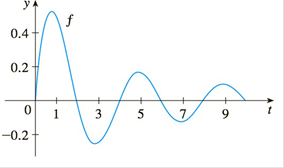Chapter 4.3, Problem 66E

Chapter
Section
Textbook Problem

# Let g ( x ) = ∫ 0 x f ( t )   d t where f is the function whosegraph is shown.(a) At what values of x do the local maximum and minimum values of g occur?(b) Where does g attain its absolute maximum value?(c) On what intervals is g concave downward?(d) Sketch the graph of g.To determine

a)

To find:

Values of x where local maximum and minimum occurs

Explanation

1) Concept:

i) If f changes from positive to negative at c, then f has a local maximum at c.

ii) If f changes from negative to positive at c, then f has a local minimum at c.

iii) If f is positive to the left and right of c or negative to the left and right of c, then f has no local maximum and minimum at c

2) Calculation:

By fundamental theorem,

g'x=f(x)

To determine

b)

To find:

The absolute maximum value

To determine

c)

To find:

On what interval is the curve concave downward

To determine

d)

To draw:

Graph of g

### Still sussing out bartleby?

Check out a sample textbook solution.

See a sample solution

#### The Solution to Your Study Problems

Bartleby provides explanations to thousands of textbook problems written by our experts, many with advanced degrees!

Get Started

#### Find more solutions based on key concepts